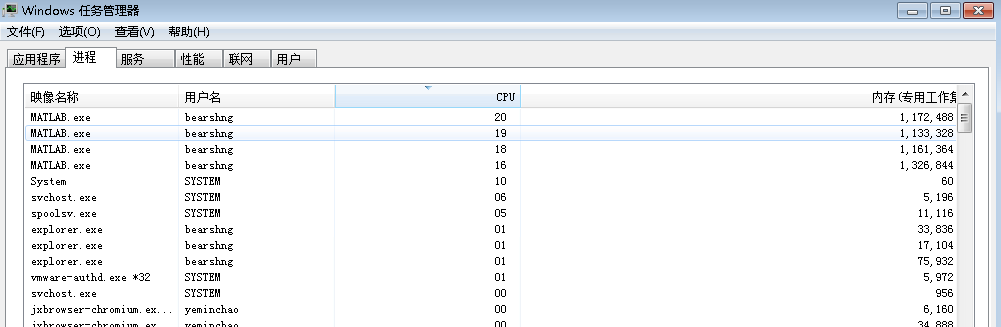## parfor的简介

parfor就是paralle+for，也就是并行的for循环，它的大致意思是会给你自动构造几个matlab的执行进程，并行地处理你的数据。这里的数目最大的数值是你的CPU的核数，比如说楼主的电脑是四核的，在任务管理器里面就看到了4个matlab的进程。1. 大量的简单计算的循环。
2. 大量或少量的复杂计算的循环
3. 各个任务之间不会出现数据的依赖,比如说循环内部的变量之间不要存在数据传递等等。

## parfor的使用

	parfor i = 1:length(A)
B(i) = f(A(i));
end


## 在parfor里面保存数据文件

Matlab默认是不允许在parfor里面使用save函数的,这个是因为

Transparency is violated by the SAVE command because in general MATLAB cannot determine which variables from

the workspace will be saved to a file.

### 使用parsave保存数据

	function parfor_save(varargin)
fname=varargin{1};
for i=2:nargin
eval([inputname(i),'=varargin{i};']);
if i==2
save('-mat',fname,inputname(i));
else
save('-mat',fname,inputname(i),'-append');
end
end


	parfor ii = 1:4
x = rand(10,10);
y = ones(1,3);
parsave(sprintf('output%d.mat', ii), x, y);
end


Error using parsave (line 27)

Transparency violation error.

See Parallel Computing Toolbox documentation about Transparency

### 自己实现save函数进行数据保存

matfile Save and load parts of variables in MAT-files.

MATOBJ = matfile(FILENAME) constructs an object that can load or save

parts of variables in MAT-file FILENAME. MATLAB does not load any data

from the file into memory when creating the object. FILENAME can

include a full or partial path, otherwise matfile searches along the

MATLAB path. If the file does not exist, matfile creates the file on

the first assignment to a variable.

MATOBJ = matfile(FILENAME,’Writable’,ISWRITABLE) enables or disables

write access to the file. ISWRITABLE is logical TRUE (1) or FALSE (0).

By default, matfile opens existing files with read-only access, but

creates new MAT-files with write access.

Access variables in MAT-file FILENAME as properties of MATOBJ, with dot

part of variable VARNAME into variable SMALLERVAR is

SMALLERVAR = MATOBJ.VARNAME(INDICES)

Similarly, the syntax for saving NEWDATA into variable VARNAME is

MATOBJ.VARNAME(INDICES) = NEWDATA

Specify part of a variable by defining indices for every dimension.

Indices can be a single value, an equally spaced range of increasing

values, or a colon (:), such as:

MATOBJ.VARNAME(100:500, 200:600)

MATOBJ.VARNAME(:, 501:1000)

MATOBJ.VARNAME(1:2:1000, 80)

	parfor ii = 1:4
m=matfile(sprintf('output%d.mat', ii),'writable',true)
x = rand(10,10);
y = ones(1,3);
m.x=x;
m.y=y;
end